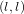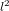Hostname: page-component-797576ffbb-k7d4m Total loading time: 0 Render date: 2023-12-04T10:31:42.568Z Has data issue: false Feature Flags: { "corePageComponentGetUserInfoFromSharedSession": true, "coreDisableEcommerce": false, "useRatesEcommerce": true } hasContentIssue false

# Computing functions on Jacobians and their quotients

Published online by Cambridge University Press:  01 August 2015

## Abstract

Core share and HTML view are not possible as this article does not have html content. However, as you have access to this content, a full PDF is available via the ‘Save PDF’ action button.

We show how to efficiently evaluate functions on Jacobian varieties and their quotients. We deduce an algorithm to compute$(l,l)$ isogenies between Jacobians of genus two curves in quasi-linear time in the degree$l^{2}$.

Type
Research Article
Information

## References

Arbarello, E., Cornalba, M., Griffiths, P. A. and Harris, J., Geometry of algebraic curves, vol. I , Grundlehren der Mathematischen Wissenschaften 267 (Springer, New York, 1985).Google Scholar
Baker, H. F., Abel’s theorem and the allied theory, including the theory of the theta functions (Cambridge University Press, Cambridge, 1897).Google Scholar
Birkenhake, C. and Lange, H., Complex abelian varieties , 2nd edn, Grundlehren der Mathematischen Wissenschaften 302 (Springer, Berlin, 2004).Google Scholar
Bostan, A., Morain, F., Salvy, B. and Schost, É., ‘Fast algorithms for computing isogenies between elliptic curves’, Math. Comp. 77 (2008) no. 263, 17551778.Google Scholar
Brent, R. P. and Kung, H. T., ‘Fast algorithms for manipulating formal power series’, J. Assoc. Comput. Mach. 25 (1978) no. 4, 581595.Google Scholar
Bürgisser, P., Clausen, M. and Amin Shokrollahi, M., Algebraic complexity theory , Grundlehren der Mathematischen Wissenschaften 315 (Springer, Berlin, 1997) ; with the collaboration of Thomas Lickteig.Google Scholar
Cosset, R. and Robert, D., ‘Computing (l, l)-isogenies in polynomial time on Jacobians of genus 2 curves’, Math. Comp. 84 (2015) 19531975.Google Scholar
Couveignes, J.-M., ‘Linearizing torsion classes in the Picard group of algebraic curves over finite fields’, J. Algebra 321 (2009) no. 8, 20852118.Google Scholar
Couveignes, J.-M., ‘Computing V f modulo p ’, Computational aspects of modular forms and Galois representations , Annals of Mathematics Studies 176 (Princeton University Press, Princeton, NJ, 2011) 337370.Google Scholar
Couveignes, J.-M. and Edixhoven, B., ‘First description of the algorithms’, Computational aspects of modular forms and Galois representations , Annals of Mathematics Studies 176 (Princeton University Press, Princeton, NJ, 2011) 6978.Google Scholar
Diem, C., ‘On arithmetic and the discrete logarithm problem in class groups of curves’, Habilitation Thesis, Leipzig, 2008.Google Scholar
Dolgachev, I. and Lehavi, D., ‘On isogenous principally polarized abelian surfaces’, Curves and abelian varieties , Contemporary Mathematics 465 (American Mathematical Society, Providence, RI, 2008) 5169.Google Scholar
Elkies, N. D., ‘Elliptic and modular curves over finite fields and related computational issues’, Computational perspectives on number theory (Chicago, IL, 1995) , AMS/IP Studies in Advanced Mathematics 7 (American Mathematical Society, Providence, RI, 1998) 2176.Google Scholar
Fay, J. D., Theta functions on Riemann surfaces , Lecture Notes in Mathematics 352 (Springer, Berlin, 1973).Google Scholar
Le Gall, F., ‘Powers of tensors and fast matrix multiplication’, ISSAC 2014 Proceedings of the 39th International Symposium on Symbolic and Algebraic Computation (ACM, New York, 2014) 296303.Google Scholar
Ghorpade, S. R. and Lachaud, G., ‘Étale cohomology, Lefschetz theorems and number of points of singular varieties over finite fields’, Mosc. Math. J. 2 (2002) no. 3, 589631; dedicated to Yuri I. Manin on the occasion of his 65th birthday.Google Scholar
Hess, F., ‘Computing Riemann–Roch spaces in algebraic function fields and related topics’, J. Symbolic Comput. 33 (2002) no. 4, 425445.Google Scholar
Joux, A. and Lercier, R., ‘Counting points on elliptic curves in medium characteristic’. Cryptology ePrint Archive, Report 2006/176, 2006.Google Scholar
Khuri-Makdisi, K., ‘Asymptotically fast group operations on Jacobians of general curves’, Math. Comp. 76 (2007) no. 260, 22132239.Google Scholar
Lachaud, G. and Martin-Deschamps, M., ‘Nombre de points des jacobiennes sur un corps fini’, Acta Arith. 56 (1990) no. 4, 329340.Google Scholar
Lang, S., Abelian varieties (Springer, New York, Berlin, 1983) ; reprint of the 1959 original.Google Scholar
Lercier, R. and Morain, F., ‘Algorithms for computing isogenies between elliptic curves’, Computational perspectives on number theory: proceedings of a conference in honor of A. O. L. Atkin , AMS/IP Studies in Advanced Mathematics 7 (eds Buell, D. A. and Teitelbaum, J. T.; American Mathematical Society & International Press, Providence, RI, 1998) 7796. Held in 1995 at the University of Illinois at Chicago.Google Scholar
Lubicz, D. and Robert, D., ‘Computing isogenies between abelian varieties’, Compos. Math. 148 (2012) no. 5, 14831515.Google Scholar
Lubicz, D. and Robert, D., ‘Computing separable isogenies in quasi-optimal time’, LMS J. Comput. Math. 18 (2015) no. 1, 198216.Google Scholar
Mascot, N., ‘Computing modular Galois representations’, Rend. Circ. Mat. Palermo (2) 62 (2013) no. 3, 451476.Google Scholar
Miller, V. S., ‘The Weil pairing, and its efficient calculation’, J. Cryptology 17 (2004) no. 4, 235261.Google Scholar
Milne, J. S., ‘Abelian varieties’, Arithmetic geometry (Springer, New York, 1986) 103150.Google Scholar
Mumford, D., Tata lectures on theta. III, Modern Birkhäuser classics (Birkhäuser Boston, Inc., Boston, MA, 2007) ; with collaboration of Madhav Nori and Peter Norman. Reprint of the 1991 original.Google Scholar
D. Mumford  Abelian varieties, Tata Institute of Fundamental Research Studies in Mathematics 5 (Published for the Tata Institute of Fundamental Research, Bombay, 2008); with appendices by C. P. Ramanujam and Y. Manin. Corrected reprint of the second 1974 edition.Google Scholar
Schoof, R., ‘Counting points on elliptic curves over finite fields’, J. Théor. Nombres Bordeaux 7 (1995) no. 1, 219254; Les Dix-huitièmes Journées Arithmétiques (Bordeaux, 1993).Google Scholar
Shepherd-Barron, N., ‘Thomae’s formulae for non-hyperelliptic curves and spinorial square roots of theta-constants on the moduli space of curves’, Preprint, 2008, arXiv:0802.3014.Google Scholar
Smith, B., ‘Computing low-degree isogenies in genus 2 with the Dolgachev–Lehavi method’, Arithmetic, geometry, cryptography and coding theory , Contemporary Mathematics 574 (American Mathematical Society, Providence, RI, 2012) 159170.Google Scholar
The PARI Group, Bordeaux. PARI/GP version 2.7.2, 2014, available fromhttp://pari.math.u-bordeaux.fr/.Google Scholar
Vélu, J., ‘Isogénies entre courbes elliptiques’, C. R. Acad. Sci. Paris 273 (1971) A238A241.Google Scholar
Vélu, J., ‘Courbes elliptiques munies d’un sous-groupe Zn Z ×𝜇 n ’, Bull. Soc. Math. France Mém. 57 (1978) 5152.Google Scholar
Weil, A., ‘Sur les courbes algébriques et les variétés qui s’en déduisent’, Actualités Sci. Ind., no. 1041 , Publ. Inst. Math. Univ. Strasbourg 7, 1945 (Hermann et Cie, Paris, 1948).Google Scholar
Weil, A., ‘Variétés abéliennes et courbes algébriques’, Actualités Sci. Ind., no. 1064 , Publ. Inst. Math. Univ. Strasbourg 8, 1946 (Hermann & Cie, Paris, 1948).Google Scholar